• ## C++随机数

万次阅读 多人点赞 2018-10-07 19:20:19
c++随机数产生以及扩展
注：本文的知识来自网络和个人的整理和理解。
当初写这篇博客的时候，用的IDE是Dev C++。当时可以直接使用诸如rand()、srand()等函数。现在好像不行了，还是需要加上#include<cstdlib>。本文的代码已经修改了，图片没法修改，见谅。
一、rand函数。
int rand(void);
rand()函数不需要引入任何头文件，可直接使用，返回0~RAND_MAX（32767）的整数，不需要参数，它是根据种子生成的，根据不同的种子产生不同的随机序列。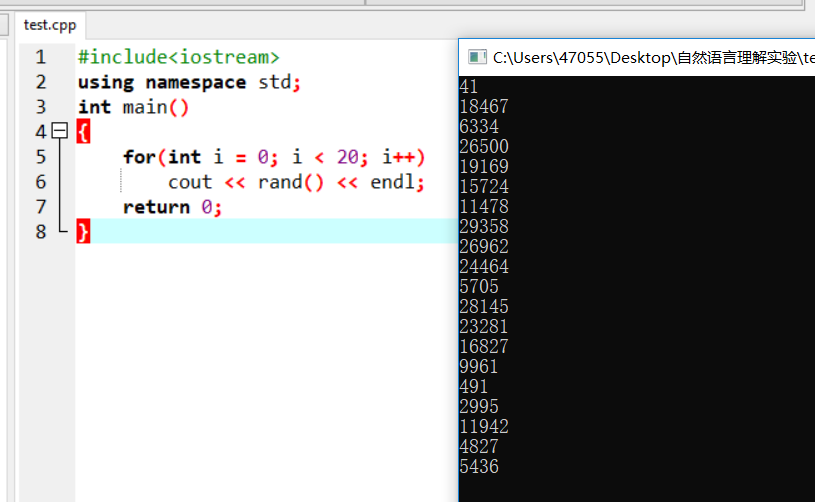这个程序无论何时何地，运行的结果都如上所示。系统默认的种子是1，随机序列又是根据种子产生的，所以说每次使用的随机序列都是固定的，随机数不随机。要想使它随机，就必须使它的种子随机。
二、srand函数。
void srand(unsigned  int  seed);
srand()函数就是用来设置rand()函数的种子的。
同样srand()函数也不需要头文件，可以直接使用，根据不同的参数产生不同的种子。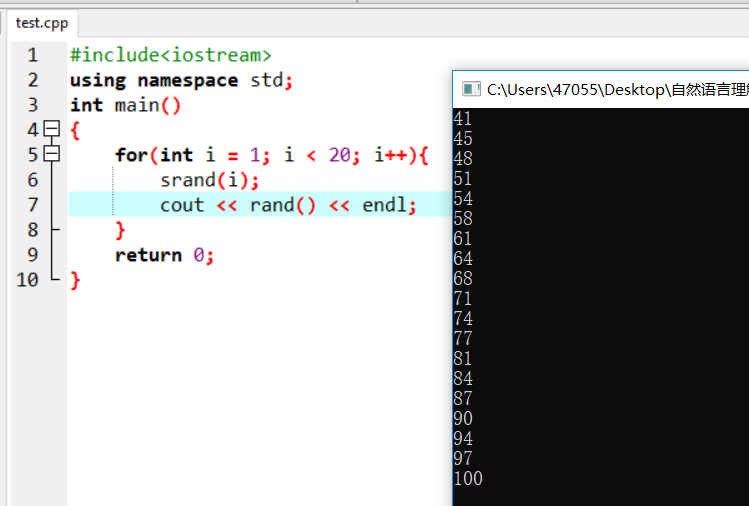同样，这个程序如同上个程序，何时何地运行结果都一样，因为srand()的参数一样，生成的种子一样，rand()函数返回的值也一样。那么就只有在srand()的参数上做文章。
三、time函数
time(NULL);
函数会返回1970年1月1日至今所经历的时间（以秒为单位），需要引入头文件<time.h>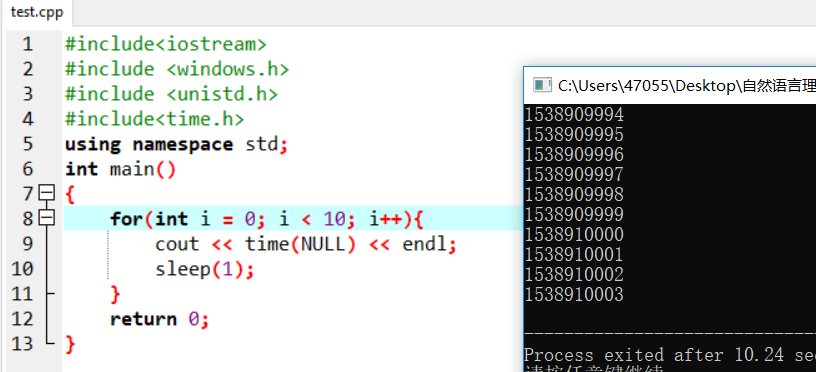用时间值做种子，就可以产生随机数了，因为时间总是在变的嘛。
将time(NULL)作为srand()的参数，更新种子，再用rand()函数产生随机数。
#include<iostream>
#include<cstdlib>
#include<time.h>
using namespace std;
int main()
{
srand(time(NULL));
cout << rand() << endl;
return 0;
}

四、注意
需要注意的是，srand(time(NULL));只需要执行一次即可。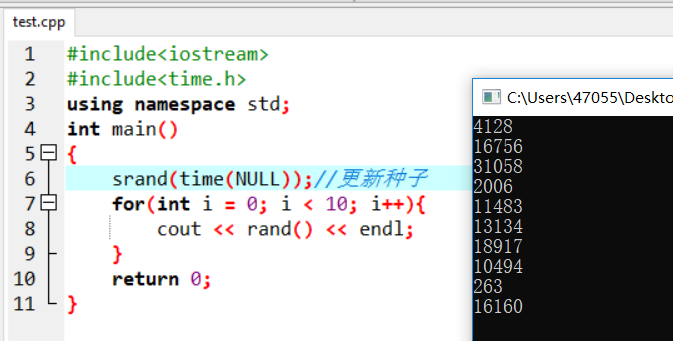如果每次使用rand()前都执行一遍，就会出现下面的情况：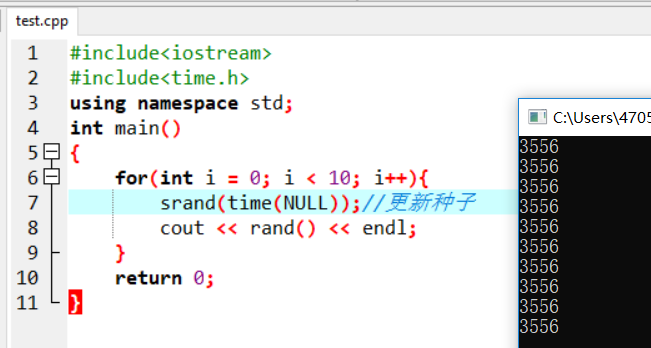这是因为time()是以秒为单位的，在同一秒内返回的值都是相同的，种子是相同的，产生的随机序列自然也相同。每次都从同一个序列的开头取数，可不就一样了嘛。
五、扩展
rand()产生的数是0~RAND_MAX( 0x7fff)，也就是说，只能生成15位。如果需要32位的，可以进行扩展：
unsigned int rand_32()
{
return (rand()&0x3)<<30 | rand()<<15 | rand();
}

扩展之后测试一下：
#include<iostream>
#include<time.h>
#include<cstdlib>
#include<vector>
using namespace std;
unsigned int rand_32()
{
return (rand()&0x3)<<30 | rand()<<15 | rand();
}
int main()
{
srand(time(NULL));
unsigned a;
for(int i = 0; i < 10; i++){
a = rand_32();
cout << hex << a << endl;
}
return 0;
}

以十六进制输出的结果如下: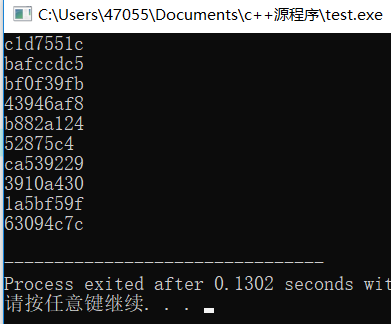因为担心这样扩展会不会出现问题，于是进行下列测试：
int main()
{
srand(time(NULL));
vector<unsigned int> v;
unsigned a;
for(long long  i = 0; i < 50000; i++){
a = rand_32();
if(a == 0)
cout << "出现 0 " << endl;
auto t = find(v.begin(),v.end(),a);
if(t != v.end()){
cout << "重复" << endl;
}
else
v.push_back(a);
}
return 0;
}

每次测试50000个数，测了三次，运行结果如下：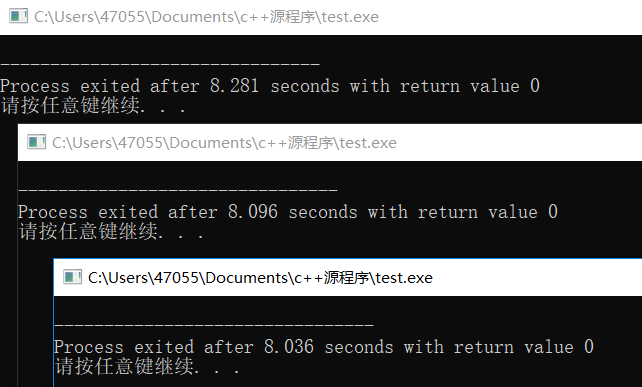没什么问题，测了三次，每次不出现0，不出现重复的值。
顺便贴上8位的代码：
unsigned char rand_8()
{
return (unsigned char)(rand()&0xff);
}



展开全文• C++随机数
#include <iostream>
#include <time.h>
using namespace std;

// 适合double类型
//template <class T>
//class Rand
//{
//	public:
//		Rand(T dMin,T dMax);
//		T GetValues() const { return dVal; };
//	private:
//		T dVal;
//};
//template <class T> Rand<T>::Rand(T dMin,T dMax)
//{
//	// srand()函数产生一个以当前时间开始的随机种子
//	srand((unsigned)time(NULL));
//	T dRatio=(T)rand()/RAND_MAX;
//	dVal= dMin + dRatio * (dMax - dMin);
//}
// 用法示例
// Rand<int > c(5,10);
// cout<<c.GetValues()<<endl;

// double (nMin,nMax)
double Rand(double dMin,double dMax)
{
double dRatio=(double)rand()/RAND_MAX;
return dMin + dRatio * (dMax - dMin);
}
// int [nMin,nMax)
int Rand(int nMin,int nMax)
{
return nMin + rand()%(nMax - nMin);
}

int main()
{
// srand()函数产生一个以当前时间开始的随机种子
srand( (unsigned)time(NULL) );
for(int i=0;i<10;i++)
{
cout<<"Rand(-10.0,10.0)("<<i+1<<") :"<<Rand(-10.0,10.0)<<endl;
}
for(int i=0;i<10;i++)
{
cout<<"Rand(-10,10)("<<i+1<<") :"<<Rand(-10,10)<<endl;
}
system("pause");
return 0;
}
展开全文• c++随机数生成函数rand()，实质生成伪随机数列。 为生成更加随机的数列，需要srand(unsigned num)来播种。 常用方式srand((unsigned)time(NULL));//增加头文件 生成[a,b]之间的随机整数的方法： 1、rand...
c++随机数生成函数rand()，实质生成伪随机数列。

为生成更加随机的数列，需要srand(unsigned num)来播种。

常用方式srand((unsigned)time(NULL));//增加头文件<time.h>

生成[a,b]之间的随机整数的方法：

1、rand()%(b-a+1)+a;

2、a+b*rand()/RAND_MAX;

两篇引用博文：第一篇讲了基本的方法，第二篇讲了一些限制条件。其实对于第二篇的情况，在随机数的范围有限的情况下，不等概率的影响是可以忽略不计的，但是当随机数区间长度很大时候，上述问题就变得比较有意义了。特别是当区间长度大于RAND_MAX的一半以上时，这种情况就需要认真处理了。

计算机的随机数都是由伪随机数，即是由小M多项式序列生成的，其中产生每个小序列都有一个初始值，即随机种子。（注意： 小M多项式序列的周期是65535，即每次利用一个随机种子生成的随机数的周期是65535，当你取得65535个随机数后它们又重复出现了。）

我们知道rand()函数可以用来产生随机数，但是这不是真正意义上的随机数，是一个伪随机数，是根据一个数（我们可以称它为种子）为基准以某个递推公式推算出来的一系列数，当这系列数很大的时候，就符合正态公布，从而相当于产生了随机数，但这不是真正的随机数，当计算机正常开机后，这个种子的值是定了的，除非你破坏了系统。

1.rand()

功能:随机数发生器

用法:int rand(void)

所在头文件: stdlib.h

rand()的内部实现是用线性同余法做的，它不是真的随机数，因其周期特别长，故在一定的范围里可看成是随机的。

rand()返回一随机数值的范围在0至RAND_MAX 间。RAND_MAX的范围最少是在32767之间(int)。用unsigned int 双字节是65535，四字节是4294967295的整数范围。0~RAND_MAX每个数字被选中的机率是相同的。

用户未设定随机数种子时，系统默认的随机数种子为1。

rand()产生的是伪随机数字，每次执行时是相同的;若要不同,用函数srand()初始化它。

2.srand()

功能:初始化随机数发生器

用法: void srand(unsigned int seed)

所在头文件: stdlib.h

srand()用来设置rand()产生随机数时的随机数种子。参数seed必须是个整数，如果每次seed都设相同值，rand()所产生的随机数值每次就会一样。

3.使用当前时钟作为随机数种子

rand()产生的随机数在每次运行的时候都是与上一次相同的。若要不同,用函数srand()初始化它。可以利用srand((unsigned int)(time(NULL))的方法，产生不同的随机数种子，因为每一次运行程序的时间是不同的。

4.产生随机数的用法
1) 给srand()提供一个种子，它是一个unsigned int类型；
2) 调用rand()，它会根据提供给srand()的种子值返回一个随机数(在0到RAND_MAX之间)；
3) 根据需要多次调用rand()，从而不间断地得到新的随机数；
4) 无论什么时候，都可以给srand()提供一个新的种子，从而进一步“随机化”rand()的输出结果。

0~RAND_MAX之间的随机数程序

#include <iostream>
#include <stdlib.h>
#include <time.h> using namespace std; int main()
{
srand((unsigned)time(NULL));
for(int i
= 0; i < 10;i++ )
cout << rand() << '\t';
cout << endl;
return 0;
}

5.产生一定范围随机数的通用表示公式
要取得[a,b)的随机整数，使用(rand() % (b-a))+ a;
要取得[a,b]的随机整数，使用(rand() % (b-a+1))+ a;
要取得(a,b]的随机整数，使用(rand() % (b-a))+ a + 1;
通用公式:a + rand() % n；其中的a是起始值，n是整数的范围。
要取得a到b之间的随机整数，另一种表示：a + (int)b * rand() / (RAND_MAX + 1)。
要取得0～1之间的浮点数，可以使用rand() / double(RAND_MAX)。

如果让你用C++来生成0——N-1之间的随机数，你会怎么做？你可能会说，很简单，看：

srand( (unsigned)time( NULL ) );
rand() % N;

仔细想一下，这个结果是随机的吗（当然，我们不考虑rand()函数的伪随机性）？

不是的，因为rand()的上限是RAND_MAX，而一般情况下，RAND_MAX并不是N的整数倍，那么如果RAND_MAX % = r，则0——r之间的数值的概率就要大一些，而r+1——N-1之间的数值的概率就要小一些。还有，如果N > RAND_MAX，那该怎么办？

下面给出一种比较合适的方案，可以生成任意范围内的等概率随机数 result。最后还有一个更简单的方法。

1、如果N<RAND_MAX+1，则要去除尾数，
R = RAND_MAX-(RAND_MAX+1)%N; //去除尾数
t = rand();
while( t > R ) t = rand();
result = t % N; // 符合要求的随机数
2、如果 N>RAND_MAX，可以考虑分段抽样，分成[n/(RNAD_MAX+1)]段，先等概率得到段再得到每段内的某个元素，这样分段也类似地有一个尾数问题，不是每次都刚好分到整数段，一定或多或少有一个余数段，这部分的值如何选取？
选到余数段的数据拿出来选取，先进行一次选到余数段概率的事件发生，然后进行单独选取：
r = N % (RAND_MAX+1); //余数
if ( happened( (double)r/N ) )//选到余数段的概率
result = N-r+myrandom(r); // myrandom可以用情况1中的代码实现
else
result = rand()+myrandom(N/(RAND_MAX+1))*(RAND_MAX+1); // 如果选不到余数段再进行分段选取

完整的代码：
#include<iostream.h>
#include<time.h>
#include<stdlib.h>
const double MinProb=1.0/(RAND_MAX+1);
bool happened(double probability)//probability 0~1
{
if(probability<=0)
{
return false;
}
if(probability<MinProb)
{
return rand()==0&&happened(probability*(RAND_MAX+1));
}
if(rand()<=probability*(RAND_MAX+1))
{
return true;
}
return false;
}

long myrandom(long n)//产生0~n-1之间的等概率随机数
{
t=0;
if(n<=RAND_MAX)
{
long R=RAND_MAX-(RAND_MAX+1)%n;//尾数
t = rand();
while ( t > r )
{
t = rand();
}
return t % n;
}
else
{
long r = n%(RAND_MAX+1);//余数
if( happened( (double)r/n ) )//取到余数的概率
{
return n-r+myrandom(r);
}
else
{
return rand()+myrandom(n/(RAND_MAX+1))*(RAND_MAX+1);
}
}
}

还有另外一种非常简单的方式，那就是使用

random_shuffle( RandomAccessIterator _First, RandomAccessIterator _Last ).

例如，生成0——N-1之间的随机数，可以这么写

#include <algorithm>
#include <vector>

long myrandom( long N )
{
std::vector<long> vl( N ); // 定义一个大小为N的vector
for ( long i=0; i<N; ++i )
{
vl[i] = i;
}

std::random_shuffle( vl.begin(), vl.end() );

return (*vl.begin());
}

random_shuffle 还有一个三参数的重载版本

random_shuffle( RandomAccessIterator _First, RandomAccessIterator _Last, RandomNumberGenerator& _Rand )

第三个参数可以接受一个自定义的随机数生成器来把前两个参数之间的元素随机化。

这个方法的缺陷就是，如果只是需要一个随机数的话，当N很大时，空间消耗很大！


展开全文...

# c++随机数c++ 订阅